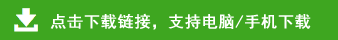# 人教版四年级上册数学综合练习题及答案（共8套）

一、直接写出下面各题得数。

8×(125－25)=　　　48＋52÷4=

160＋40÷4=　　　 (19－11)×125=

(12＋42÷7)×5=　　 26×8÷26×8=

1．(841－41)÷25×4　　　 2．600×(1200－200÷25)

=800÷25×4　　　　　　　　=600×(1000÷25)

=8　　　　　　　　　　　　=24000

1．3280÷16=205　　　　 2．23×16=368

205×10=2050　　　　　　625－368=257

6000－2050=3950　　　　1028÷257=4